# ISEE Upper Level Quantitative : How to find the area of a triangle

## Example Questions

### Example Question #1 : How To Find The Area Of A Triangle

Triangle B has a height that is twice that of Triangle A and a base that is one-half that of Triangle A. Which is the greater quantity?

(a) The area of Triangle A

(b) The area of Triangle B

It is impossible to tell from the information given.

(a) is greater.

(a) and (b) are equal.

(b) is greater.

(a) and (b) are equal.

Explanation:

Letandbe the base and height of Triangle A. Then the base and height of Triangle B are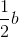and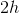, respectively.

(a) The area of Triangle A is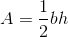.

(b) The area of Triangle B is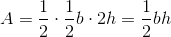.

Therefore, (a) and (b) are equal.

### Example Question #2 : How To Find The Area Of A Triangle

Two triangles on the coordinate plane have a vertex at the origin and a vertex at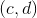, where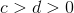.

Triangle A has its third vertex at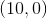.

Triangle B has its third vertex at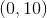.

Which is the greater quantity?

(a) The area of Triangle A

(b) The area of Triangle B

It is impossible to tell from the information given

(a) is greater

(a) and (b) are equal

(b) is greater

(b) is greater

Explanation:

(a) Triangle A has as its base the horizontal segment connecting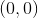and, the length of which is 10. Its (vertical) altitude is the segment fromto this horizontal segment, which is part of the-axis; its height is therefore the-coordinate of this point, orThe area of Triangle A is therefore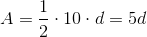(b) Triangle B has as its base the vertical segment connectingand, the length of which is 10. Its (horizontal) altitude is the segment fromto this vertical segment, which is part of the-axis; its height is therefore the-coordinate of this point, orThe area of Triangle B is therefore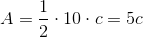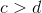, so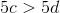. (b), the area of Triangle B, is greater.

### Example Question #3 : How To Find The Area Of A Triangle

A triangle has sides 30, 40, and 80. Give its area.

None of the other responses is correct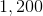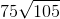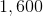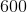None of the other responses is correct

Explanation:

By the Triangle Inequality Theorem, the sum of the lengths of the two shorter sides of a triangle must exceed the length of its longest side. However,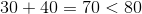;

Therefore, this triangle cannot exist, and the correct answer is "none of the other responses is correct".

### Example Question #4 : How To Find The Area Of A Triangle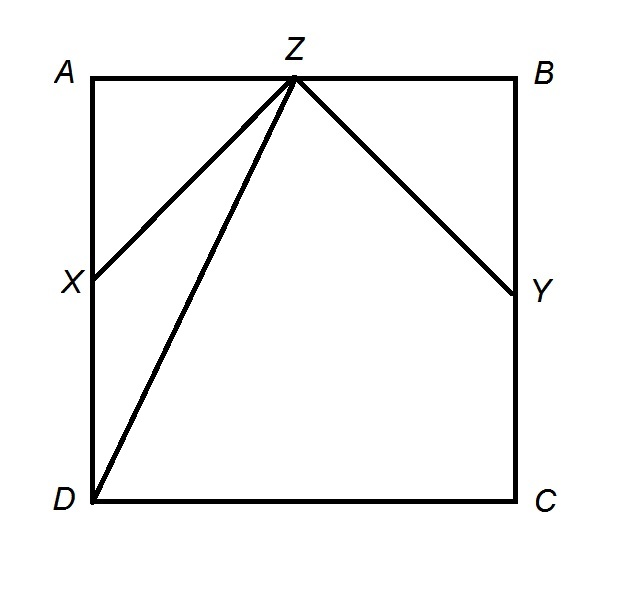The above depicts Square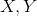, and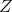are the midpoints of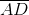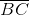, and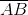, respectively. Which is the greater quantity?

(a) The area of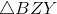(b) The area of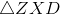(b) is the greater quantity

It is impossible to determine which is greater from the information given

(a) is the greater quantity

(a) and (b) are equal

(a) and (b) are equal

Explanation:

For the sake of simplicity, assume that the square has sidelength 2; this reasoning is independent of the actual sidelength.

Since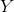, andare the midpoints of their respective sides,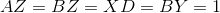, as shown in this diagram.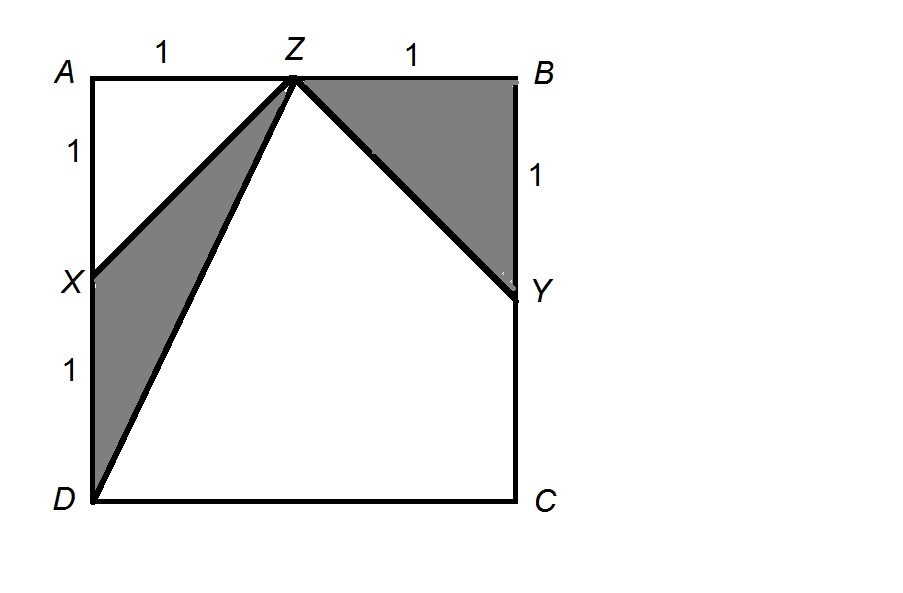The area of, it being a right triangle, is half the product of the lengths of its legs: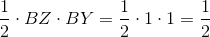The area ofis half the product of the length of a base and the height. Using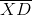as the base, and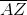as an altitude: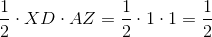The two triangles have the same area.